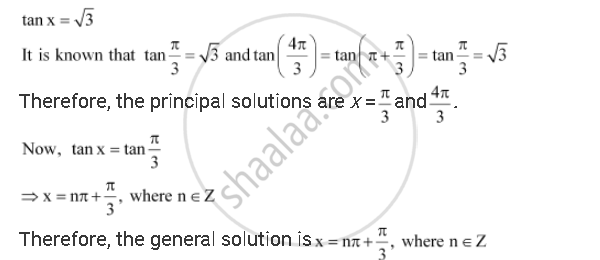CBSE (Arts) Class 11CBSE
Share

# Find the Principal and General Solutions of the Equation Tan X = Sqrt3 - CBSE (Arts) Class 11 - Mathematics

ConceptTrigonometric Equations

#### Question

Find the principal and general solutions of the equation tan x = sqrt3

#### SolutionIs there an error in this question or solution?

#### APPEARS IN

NCERT Solution for Mathematics Textbook for Class 11 (2018 to Current)
Chapter 3: Trigonometric Functions
Q: 1 | Page no. 78

#### Video TutorialsVIEW ALL 

Solution Find the Principal and General Solutions of the Equation Tan X = Sqrt3 Concept: Trigonometric Equations.
S